# Riccati equation

(diff) ← Older revision | Latest revision (diff) | Newer revision → (diff)

A first-order ordinary differential equation of the form(1)

where,andare constants. This equation was first studied by J. Riccati (1723, see ); individual cases of the equation were examined earlier. D. Bernoulli (1724–1725) established that the equation (1) can be integrated by elementary functions if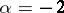or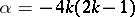, whereis an integer. J. Liouville (1841) proved that for other values ofthe solution of (1) cannot be expressed in quadratures by elementary functions. The general solution of (1) can be written with the aid of cylinder functions (see ).

The differential equation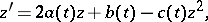(2)

where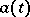,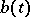and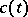are continuous functions, is called a general Riccati equation (as distinct from (1), which is called a special Riccati equation). When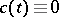, the general Riccati equation is a linear differential equation, when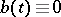, it is a Bernoulli equation. Solutions of these two equations can be found in quadratures. Other cases of the integrability of general Riccati equations have also been studied (see ).

Equation (2) is closely related to the system of differential equations(3)

If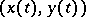is the solution of the system (3) whenand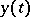does not vanish on, then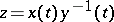is the solution of (2); ifis the solution of (2), yetis the solution of the equation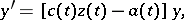then the pair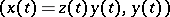is the solution of (3). In particular, solutions of (2) when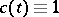are related to solutions of the equation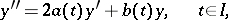by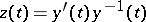ifdoes not vanish on. Because of this relation, the equation (2) is often drawn upon for the study of oscillation, non-oscillation (cf. Oscillating differential equation), reducibility (cf. Reducible linear system), and many other questions concerning the qualitative behaviour of linear equations and second-order systems (see , ).

The equation(4)

where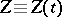is an unknown-matrix-function, and the matrix-functions,,, andhave dimensions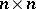,,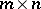, and, respectively, is called a matrix Riccati equation. The solution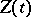of the matrix Riccati equation (4) is related to the solutionsof the linear matrix system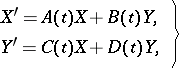(5)

by.

The matrix Riccati equation plays an important part in the theory of linear Hamiltonian systems (cf. Hamiltonian system, linear), the calculus of variations, problems of optimal control, filtration, stabilization of controllable linear systems, etc. (see Control system, and , ). For example, the optimum controlin the problem of minimization of the functional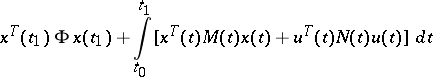on solutions of the system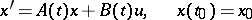(the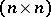-matricesand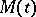are symmetric and non-negative definite, and the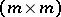-matrix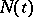is positive definite when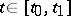), is given by the equationwhereis the solution of the matrix Riccati equation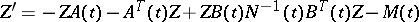(6)

with the boundary condition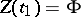(see , ).

In problems of control on an infinite time interval, the important questions concern the existence for the matrix Riccati equation of a non-negative definite solution bounded on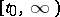, of a periodic or almost-periodic solution (in the case of periodic or almost-periodic coefficients of the equation) and the means of an approximate construction of such solutions.

In variational problems with discrete time and problems of discrete optimization the following recurrent matrix Riccati equation serves as an analogue to equation (4):Equation (4) can naturally be put into correspondence with a dynamical system on a Grassmann manifold(see ) so that the theory of dynamical systems can be applied to (4). For example, if the matrices,,, andin (4) are periodic with period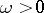and if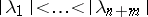, whereare the multipliers (see Floquet–Lyapunov theorem) of the system (5), then the corresponding dynamical system is generated by a Morse–Smale diffeomorphism (see Morse–Smale system), and is thus structurally stable.